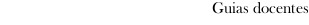Educational guide 2017_18Escola de Enxeñaría Forestal
 Grao en Enxeñaría ForestalSubjectsMathematics: Mathematics and ITContents
 Topic Sub-topic Subjet 1. The body of complex numbers The body of complex numbers. Representation of complex numbers. Module and argument. Euler's Formula. Operations with complex numbers in polar form: powers (De Moivre's formula), roots, exponentials, logarithms. Subject 2. Vectorial spaces The vectorial space Rn. Vectorial Subspaces . Linear combination. Dependency and linear independence. Vectorial spaces of finite dimension. Base and dimension. Rank. Subject 3. Linear applications Linear applications. Properties. Core and image of a linear application. Characterisation of the linear applications injectives and surjectives. Rank of a linear application. Matrix associated to a linear application. Subject 4. Matrices Definition and types of matrices. Vectorial space of the matrices mxn. Product of matrices. Regular matrix. Rank of a matrix. Calculation of the rank of a matrix and of the reverse matrix by means of elementary operations. Subject 5. Determinants Determinants Of a square matrix of order 2 and of order 3. Properties. Development by attachments. Calculation of the reverse matrix. Calculation of the rank of a matrix. Subject 6. Systems of linear equations Systems of linear equations: matrix form. Equivalent systems. Existence of solutions: theorem of Rouché-Frobenius. Homogeneous systems. Resolution of systems of linear equations: resolution by means of the methods of elimination of Gauss and Gauss-Jordan. Resolution of a system of Cramer. Resolution of a general system using the rule of Cramer. Subject 7. Euclidean vectorial space Scalar product. Norma. Distance. Orthogonality. Scalar product regarding a base. Orthogonal and orthonormal systems. Vectorial product. Mixed product. Areas and volumes. Subject 8. Geometry Three-dimensional affine space. The straight in the affine space. Equations of the straight. The plane in the affine space. Equations of the plane. Relations of incidence between straight and planes. Angles: of two straight, of two planes and of straight and plane. Distances: of a point to a plane, of a straight to a plane and of two straight that cross . Metric study of the conical. Subject 9. Diagonalization Of endomorphisms and matrices Vectors and own values. Subspaces Own. Characteristic polynomial. Diagonalization: Conditions. Polynomial nullifier. Theorem of Cayley-Hamilton. Applications. Subject 10. Convergence in R. Topology of the straight real: points distinguished, compact groups. Convergent successions in R. Operations with limits. Calculation of limits: indeterminations, rules of Stolz, of the arithmetical and geometrical averages and of the root. Numerical series. Geometrical and telescopic series. Series of positive terms. Criteria of convergence. Series alternated. Criterion of Abel. Absolute convergence. Sumation Of some elementary series. Subject 11. Limit and continuity of functions of a real variable Limit of a function in a point. Sequential limit. Properties of the limits. Calculation of limits. Continuity of real functions. Discontinuity: Types. Operations with continuous functions. Relative theorems to the global continuity: continuous image of a compact, theorem of Bolzano-Weierstrass, theorem of Bolzano: consequences. Continuity of the reverse function and of the compound function. Subject 12. Differential calculation of a variable Derived from a function in a point. Geometric interpretation of the concept of derivative. The differential. Derived function. Successive derivatives. Relationship between continuity and derivability. Calculation of derivatives: derived from the compound function and the inverse function. Theorems relating to derivable functions: Rolle's theorem, consequences; The mean value theorem, consequences; The rule of L'Hôpital, calculation of indeterminate limits. Taylor polynomials of a function. Taylor's theorem. Problems of highs and lows. Study of concavity and convexity. Turning points. Graphical representation of functions Subject 13. Integration of functions of a variable The Riemann integral: partitions, upper and lower sums, upper and lower integral, integral functions, the integral as sum limit. Properties. Theorem of the mean value. The fundamental theorem of integral calculus. Barrow Rule. Primitives. General methods of calculating primitives. Integrals improper. Geometric applications of the integral. Subject 14. Informatics Operating systems: classification, components, examples. Programming Fundamentals. Organization of archives. Methods of sorting and searching. Concept and types of databases. LABORATORY PRACTICE AGENDA Practice 1. Introduction to the syntax of a symbolic calculation program. Basic commands of a symbolic calculation program Practice 2. Complex Numbers Complex arithmetic in binomial form. Polar shape. Arithmetic in polar form Practice 3. Vector Spaces Operations with vectors. Linear independence of vectors and calculation of bases. Generator systems. Range of a vector system. Practice 4. Linear Applications Calculation of the associated matrix. Calculation of the kernel, image and rank Practice 5. Matrices and determinants Operations with matrices. Calculation of the determinant of a square matrix. Calculate the range of a matrix and the inverse matrix Practice 6. Systems of linear equations Resolution of linear systems. Cramer's Rule and Gauss and Gauss-Jordan Elimination Methods. Applications. Practice 7. Euclidean Vector Space and Geometry Calculation of the scalar product, vectorial and mixed. Calculation of areas, volumes, angles and distances. Conical curves Practice 8. Diagonalization Calculation of the eigenvalues ​​and eigenvectors of a square matrix. Diagonalization of matrices. Applications Practice 9. Convergence and Series Inheritance limit. Application of the convergence criteria of series. Sum of series. Practice 10. Functions Calculating the limit of a function at a point. Graphical representation of functions. Study of continuity. Practice 11. Derivation. Derivation of functions. Calculation of tangent and normal lines. Problems of relative extremes. Developments in Taylor series. Local study of functions. Practice 12. Integration Calculation of primitives. Applications: calculation of areas, volumes, arc lengths, moments of inertia, etc Subject 13. Informatics Programming Fundamentals. Development and management of databases
 Universidade de Vigo            | Reitoría | Campus Universitario | C.P. 36.310 Vigo (Pontevedra) | Spain | Tlf: +34 986 812 000